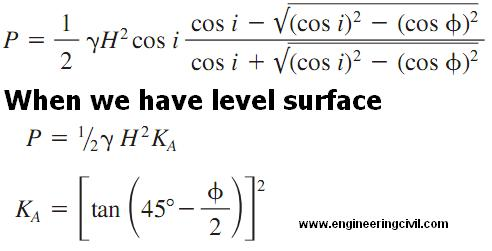Search

# What is Lateral Pressures In Cohesionless Soils?

Posted in Soil Engineering |Email This Post |

The lateral pressure in cohesion less soils is given by following formula:-

Following terms are used in the formula given below

Y=unit weight of soil, lb/ft3 or kg/m3
P=total thrust of soil, lb/linear ft (kg/m) of wall
H= total height of wall, ft (m)
KA= coefficient of active pressureMore Entries :